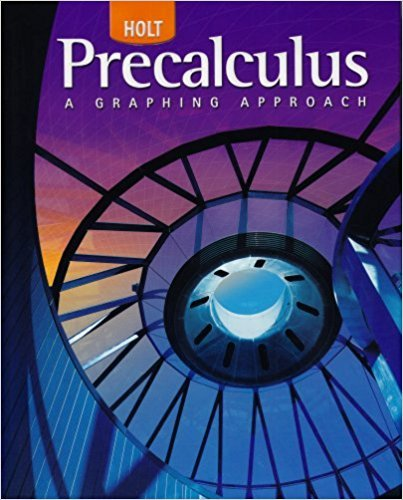×

×

# Solutions for Chapter 2.2: Solving Quadratic Equations Algebraically## Full solutions for Precalculus | 1st Edition

ISBN: 9780030416477Solutions for Chapter 2.2: Solving Quadratic Equations Algebraically

Solutions for Chapter 2.2
4 5 0 332 Reviews
11
4
##### ISBN: 9780030416477

This expansive textbook survival guide covers the following chapters and their solutions. Chapter 2.2: Solving Quadratic Equations Algebraically includes 74 full step-by-step solutions. Precalculus was written by and is associated to the ISBN: 9780030416477. Since 74 problems in chapter 2.2: Solving Quadratic Equations Algebraically have been answered, more than 54630 students have viewed full step-by-step solutions from this chapter. This textbook survival guide was created for the textbook: Precalculus, edition: 1.

Key Calculus Terms and definitions covered in this textbook
• Absolute value of a real number

Denoted by |a|, represents the number a or the positive number -a if a < 0.

P(A or B) = P(A) + P(B) - P(A and B). If A and B are mutually exclusive events, then P(A or B) = P(A) + P(B)

• Control

The principle of experimental design that makes it possible to rule out other factors when making inferences about a particular explanatory variable

• Difference of functions

(ƒ - g)(x) = ƒ(x) - g(x)

• Differentiable at x = a

ƒ'(a) exists

• Distance (in a coordinate plane)

The distance d(P, Q) between P(x, y) and Q(x, y) d(P, Q) = 2(x 1 - x 2)2 + (y1 - y2)2

• Dot product

The number found when the corresponding components of two vectors are multiplied and then summed

• Frequency table (in statistics)

A table showing frequencies.

• Fundamental

Theorem of Algebra A polynomial function of degree has n complex zeros (counting multiplicity).

• Independent events

Events A and B such that P(A and B) = P(A)P(B)

• Interval

Connected subset of the real number line with at least two points, p. 4.

• Left-hand limit of f at x a

The limit of ƒ as x approaches a from the left.

• Linear correlation

A scatter plot with points clustered along a line. Correlation is positive if the slope is positive and negative if the slope is negative

• Mathematical induction

A process for proving that a statement is true for all natural numbers n by showing that it is true for n = 1 (the anchor) and that, if it is true for n = k, then it must be true for n = k + 1 (the inductive step)

• Midpoint (on a number line)

For the line segment with endpoints a and b, a + b2

• Normal distribution

A distribution of data shaped like the normal curve.

• Rectangular coordinate system

See Cartesian coordinate system.

• Sinusoid

A function that can be written in the form f(x) = a sin (b (x - h)) + k or f(x) = a cos (b(x - h)) + k. The number a is the amplitude, and the number h is the phase shift.

• Standard unit vectors

In the plane i = <1, 0> and j = <0,1>; in space i = <1,0,0>, j = <0,1,0> k = <0,0,1>

• Tangent line of ƒ at x = a

The line through (a, ƒ(a)) with slope ƒ'(a) provided ƒ'(a) exists.